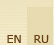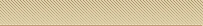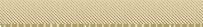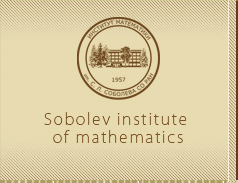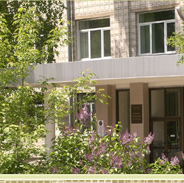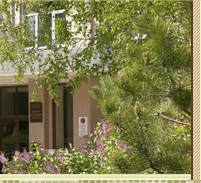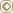MainWeb-postSite mapS. L. S o b o l e vPublicationsS e r g e i  L'v o v i c h  S o b o l e v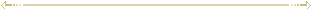Publications  (1964-1966) Lectures on the theory of cubature formulas Part I (Russian) Lectures delivered at Novosibirsk State University, 1963/64. — Novosibirsk, 1964. — 192 pp. MR0213018 (35 #3883) Cybernetics and natural sciences (Russian) // Dialectics in sciences about the abiocoen. — Мoscow, 1964. — P. 86-103. — With  A. A. Lyapunov. Decipherment of the Maya writing system (Russian) // Tr. 4 Vsesoyuzn. mat. s’’ezda. Vol. 2, — Leningrad, 1964. — P. 622. (Talk not published). Scientific search (Russian) // Za nauku v Sibiri. — 1964. — March 23. «Same radium mining». Poetry of science (Russian) // Literary Russia. — 1964. — August 14. — No. 33. — P. 10-11. Lectures on the theory of cubature formulas Part II (Russian) Lectures delivered at Novosibirsk State University, 1964/65. — Novosibirsk, 1965. — 263 pp. MR0213019 (35 #3884) Evaluation of intagrals of unboundetly differentiable functions (Russian) // Dokl. Akad. Nauk SSSR. — 1965. — Vol. 163, No. 1. — P. 33-35. MR0193406 (33 #1626) Evaluation of integrals of infinitely differentiable functions (English) // Soviet Math. Dokl. — 1965. — Vol. 6. — P. 892-894. Zbl. 147.14202 Feliks Ruvimovich Gantmakher: (Obituary) (Russian) // Uspekhi Mat. Nauk. — 1965. — Vol. 20, No. 2. — P. 149-157. — With M. A. Aizerman et al. MR0182546 (32 #29) Feliks Rubimovich Gantmakher: (Nachruf) (English) // Russ. Math. Surveys. — 1965. — Vol. 20, No. 2. — P. 143-151. — With M. A. Ajzerman et al. Zbl. 127.00604 Cubature formulas with regular boundary layer (Russian) // Dokl. Akad. Nauk SSSR. — 1965. — Vol. 163, No. 3. — P. 587-590. MR019245 (33 #472) Cubature formulas with regular boundary layer (English) // Soviet Math. Dokl. — 1965. — Vol. 6. — P. 984-987. Zbl. 147.14203 On the order of convergence of cubature formulas (Russian) // Dokl. Akad. Nauk SSSR. — 1965. — Vol. 162, No. 5. — P. 1005-1008. MR0179528 (31 #3776) On the order of convergence of cubature formulas (English) // Soviet Math. Dokl. — 1965. — Vol. 6. — P. 808-811. Zbl. 143.38706 Representation of periodic analytic functions by a sum of squares (Russian) // Dokl. Akad. Nauk SSSR. — 1965. — Vol. 165, No. 1. — P. 40-43. Representation of periodic analytic functions by a sum of squares (English) // Soviet Math. Dokl. — 1965. — Vol. 6. — P. 1412-1415. Zbl. 146.12801 MR0198296 (33 #6455) A difference analogue of a polyharmonic equation (Russian) // Dokl. Akad. Nauk SSSR. — 1965. — Vol. 164, No. 1. — P. 54-57. MR0190361 (32 #7774) A difference analog of the polyharmonic equation (English) // Soviet Math. Dokl. — 1965. — Vol. 6. — P. 1174-1178. Zbl. 142.08801 Optimal formulae of mechanical quadratures with nodes at the points of regular lattices (Russian) // Dokl. Akad. Nauk SSSR. — 1965. — Vol. 164, No. 2. — P. 281-284. MR0189247 (32 #6674) Optimal mechanical cubature formulas with interpolation points on a regular grid (English) // Soviet Math. Dokl. — 1965. — Vol. 6. — P. 1226-1229. Zbl. 142.12204 Optimierung numerischer Methoden (Russian) // Apl. Mat . — 1965. — Vol. 10, No. 2. — P. 96-129. — With I. Babushka. Zbl. 128.36204 Optimization of numerical processes (Russian) // Apl. Mat . — 1965. — Vol. 10, No. 2. — P. 96-129. — With I. Babushka. MR0191072 (32 #8481) The convergence of approximate integration formulae on functions in the class L2m (Russian) // Dokl. Akad. Nauk SSSR. — 1965. — Vol. 162, No. 6. — P. 1259-1261. MR0179529 (31 #3777) Convergence of approximate integration formulas for functions from L2m (English) // Soviet Math. Dokl. — 1965. — Vol. 6. — P. 865-867. Zbl. 143.38801 Sur une classe des problemes de physique mathematique (French) // Atti del Simpos. Intern. Appl. Analisi alla Fisica Matematica, 1964, Cagliari-Sassari. — Roma, 1965. — P. 192-208. Zbl. 142.22602 MR0223735 (36 #6783) Theorie der Kubatur-Formeln (Russian) // Wiss. Z. Hochsch. Archit. Bauwes. Weimar. — 1965. — Vol. 12. — P. 537-546. Zbl. 156.17104 The theory of cubature formulae (Russian) // Wiss. Z. Hochsch. Architektur Bauwesen Weimar. — 1965. — Vol. 12. — P. 537-546. MR0202310 (34 #2183) A new mathematical direction [in the field of mathematical cybernetics] (Russian) // Pravda. — 1965. — April 8. (Mosk. vypusk). — With  V. M. Glushkov and M. A. Lavrent’ev. Equetions of mathematical physics (Russian) Fourth edition — Moscow: Nauka, 1966. — 443 pp. MR0204873 (34 #4708) Men of Soviet science: On the 50th birthday of A.V. Bitsadze (Russian) // Differ. Uravn. — 1966. — Vol. 2, No. 5. — P. 716-719. — With A. N. Tikhonov and N. P. Erugin. Zbl. 176.27103 Zum 50. Geburtstag von A. V. Bitsadze (Russian) // Differ. Uravn. — 1966. — Vol. 2, No. 5. — P. 716-719. — With A. N. Tikhonov and N. P. Erugin. Zbl. 156.24706 To the 50th birthday of A.V. Bitsadze (Russian) // Differcialnye Uravnenija — 1966. — Vol. 2, No. 5. — P. 716-718. — With A. N. Tikhonov and N. P. Erugin. MR0192993 (33 #1215) On the density of finite functions in Lpl (Russian) // Dokl. Akad. Nauk SSSR. — 1966. — Vol. 167, No. 3. — P. 516-518. MR0203450 (34 #3301) Density of finite functions in Lpl (English) // Soviet Math. Dokl. — 1966. — Vol. 7. — P. 421-423. Zbl. 185.20505 Construction of cubature formulas with a regular boundary layer (Russian) // Dokl. Akad. Nauk SSSR. — 1966. — Vol. 166, No. 2. — P. 295-297. MR0191093 (32 #8502) Construction of cubature formulas with a regular boundary layer (English) // Soviet Math. Dokl. — 1966. — Vol. 7. — P. 80-82. Zbl. 226.65021 Theory of approximation of integrals of functions of several variables (Russian) // Trudy Mezhdunarod. Kongr. Mat. — Moscow, 1966. — P. 163-168. Don’t waste time: [On the beginning of the school year] (Russian) // Pravda. — 1966. — September 1. Masters of numbers (Russian) // Izvestiya. — 1966. — April 24. The base of discoveries (Russian) // Izvestiya. — 1966. — June 16.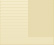©Sobolev Institute of Mathematics, 2009      Siberian Branch of the Russian Academy of Sciences4 Acad. Koptyug avenue, 630090 Novosibirsk Russia Tel.: (383) 333-28-92 Fax: (383) 333-25-98 E-mail: